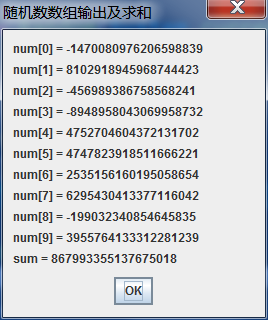# java随机数组实现怎么编写代码？

TheDisguiser 2020-08-15 15:44:00 java常见问答 4119

```import java.util.*;
import javax.swing.*;
public class SumRandom
{
public static void main(String[] args)
{
// TODO Auto-generated method stub
long num[] = new long; // 声明数组类型与大小；
String result = ""; // 存放数组内容输出结果，初值为空串
long sum = 0; // 和
Random in = new Random(System.currentTimeMillis()); // 创建Random类的对象引用in
for (int i = 0; i < 10; i++)
{
// 求和并且保存输出结果到result
num[i] = in .nextLong(); // 给数组元素分配随机数
sum += num[i]; // 求和
result += "num[" + i + "] = " + num[i] + "\n"; // 存放数组内容输出结果
}
JOptionPane.showMessageDialog(null, result + "sum = " + sum, "随机数数组输出及求和", JOptionPane.CLOSED_OPTION);
// 创建消息框进行结果输出
}
}```javascript将字符串字母转换为大小写字母

java随机数不重复有什么方法可以解决?